# Morse Code USB Keyboard Mk II

2 Nov 2015
Progress: Complete

I'm now going to eat my words from when I made my first USB Morse code key. I said that using a microcontroller was overkill. The usb-serial cable worked well enough, but it had a couple of major problems: inconvenience and total lack of portability. Can you believe that the cheap cable required a driver to work on windows? And always having to run a python script couldn't be less convenient, not to mention it took up 10%-ish of your CPU when left running in the background. I used it for a while, but when I got a new laptop I just couldn't be bothered to set it up again.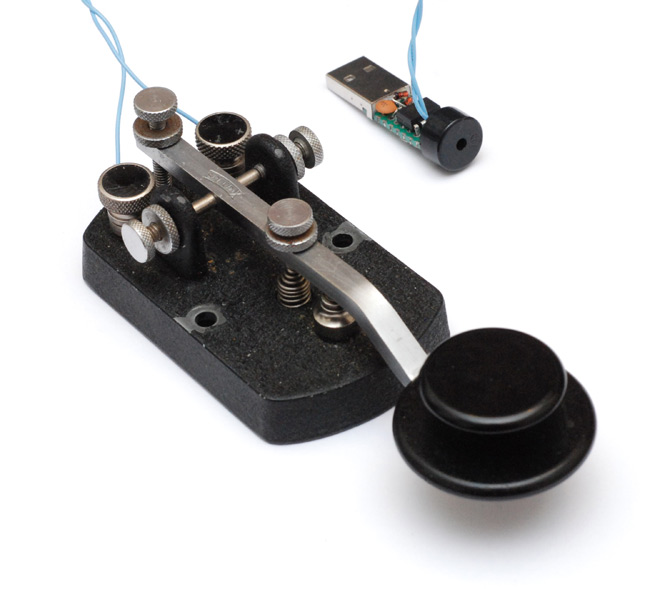I'd been wanting to make something with USB for a while anyway. I spent a few hours reading through the USB specification but I just couldn't cope. I was planning on bit-banging it in assembler. Finally I gave up, bit the bullet, and used the V-USB library. This, once I finally got it to compile, was shockingly easy to use. The disappointing part is I still don't really understand USB at all.

The most important aspect of this project is being a 'Human Interface Device' that doesn't require a driver. It presents itself as a USB keyboard. This means you can plug it into any computer and it works without a fuss. In addition, all of the processing is done by the chip, so there's no reason not to leave it plugged in all the time. When you connect it to a machine for the first time, you get a glorious message like this: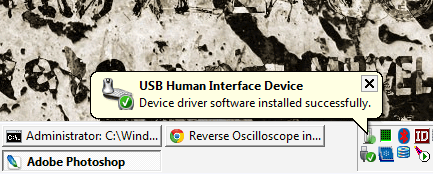The code is based on an example from the V-USB website called EasyLogger. It reads the ADC and emulates a keyboard to type the value. I essentially just translated my original script into C and squeezed it in. It uses the same character set as before, but one thing I added was holding down the key for much longer than the dash length triggers a backspace. The code is not beautiful or clever, but it's perfectly functional and was written extremely quickly. I started the project at about 3pm on Saturday, and I had to go out at 6pm, so I set myself that as a deadline and just got it finished in time.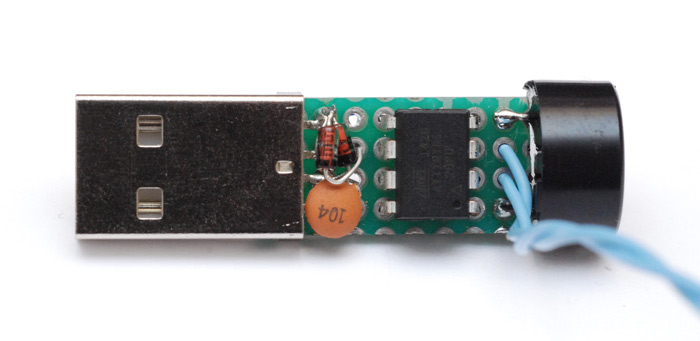I left room around the chip to fit a test clip onto it and reprogram as needed. Currently that's the only way of adjusting the speed, although the current values do have a very wide tolerance. There's one pin unused, perhaps I could squeeze a trimpot onto it for external adjustment. Heh... the ATtiny85 was 90p, the USB connector, the piezo and the passives probably add up to 50p -- astoundingly, the whole thing cost less than the usb-serial cable of the first version.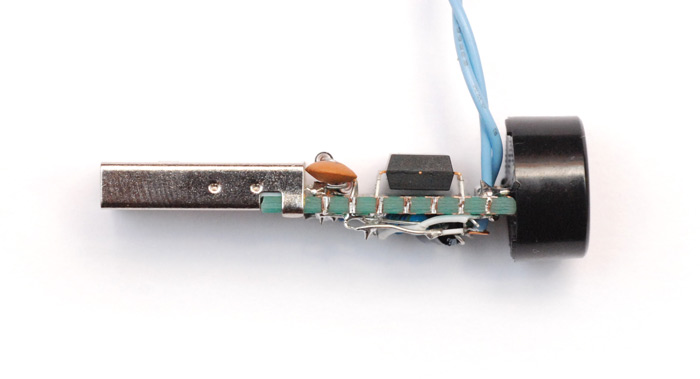Bit messy but I was soldering at breakneck speed.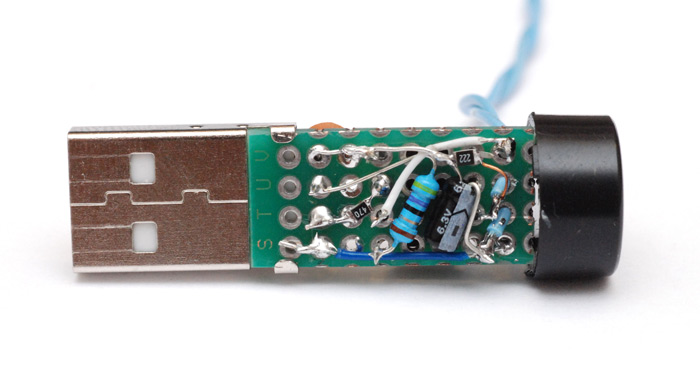Instead of a dictionary lookup for working out what was typed, I used one long string of comparisons. Tedious as it was to write it out like this, it was made much easier by this diagram from wikipedia. It also runs quite quick, as most characters can be identified in fewer than five comparisons. The keycodes I found here.If you want to recreate this, the easiest way is to download EasyLogger and replace the main.c file with the code below. Alternatively, the compiled hex file is available here. (Remember to set the low fuse to C1 for the PLL clock source.) The project uses the example vendor and hardware IDs provided by Objective Development. There are a number of rules regarding the use of these, but I didn't pay too much attention since this is only for personal use.

Update (April 2018): The hex file linked to above may have been incorrect, I have fixed the link. The complete source code is also now available on github.

```/* Morse Code USB Keyboard
* based on EasyLogger from Objective Development
* mitxela.com/projects/morse_code_usb_keyboard_mk_ii
*/

#include <avr/io.h>
#include <avr/wdt.h>
#include <avr/eeprom.h>
#include <avr/interrupt.h>
#include <avr/pgmspace.h>
#include <util/delay.h>

#include "usbdrv.h"

/*
Pin assignment:
PB1 = waveform output to piezo
PB4 = key input (active low with pull-up)

PB0, PB2 = USB data lines
*/

#define BIT_PIEZO 1
#define BIT_KEY 4

#define UTIL_BIN4(x)        (uchar)((0##x & 01000)/64 + (0##x & 0100)/16 + (0##x & 010)/4 + (0##x & 1))
#define UTIL_BIN8(hi, lo)   (uchar)(UTIL_BIN4(hi) * 16 + UTIL_BIN4(lo))

#ifndef NULL
#define NULL    ((void *)0)
#endif

/* ------------------------------------------------------------------------- */

const uchar     dashlength  = 9;
const uchar     spacelength = 45;   // longer for farnsworth pause

static uchar    reportBuffer;    // buffer for HID reports
static uchar    idleRate;           // in 4 ms units

static uchar    modifier;

static uchar    valueBuffer;
static uchar    *nextDigit;

static uchar    symbolBuffer;
static uchar    *symbol;

/* ------------------------------------------------------------------------- */

const PROGMEM char usbHidReportDescriptor[USB_CFG_HID_REPORT_DESCRIPTOR_LENGTH] = { /* USB report descriptor */
0x05, 0x01,                 // USAGE_PAGE (Generic Desktop)
0x09, 0x06,                 // USAGE (Keyboard)
0xa1, 0x01,                 // COLLECTION (Application)
0x05, 0x07,                 // USAGE_PAGE (Keyboard)
0x19, 0xe0,                 // USAGE_MINIMUM (Keyboard LeftControl)
0x29, 0xe7,                 // USAGE_MAXIMUM (Keyboard Right GUI)
0x15, 0x00,                 // LOGICAL_MINIMUM (0)
0x25, 0x01,                 // LOGICAL_MAXIMUM (1)
0x75, 0x01,                 // REPORT_SIZE (1)
0x95, 0x08,                 // REPORT_COUNT (8)
0x81, 0x02,                 // INPUT (Data,Var,Abs)
0x95, 0x01,                 // REPORT_COUNT (1)
0x75, 0x08,                 // REPORT_SIZE (8)
0x25, 0x65,                 // LOGICAL_MAXIMUM (101)
0x19, 0x00,                 // USAGE_MINIMUM (Reserved (no event indicated))
0x29, 0x65,                 // USAGE_MAXIMUM (Keyboard Application)
0x81, 0x00,                 // INPUT (Data,Ary,Abs)
0xc0                        // END_COLLECTION
};
/* We use a simplified keyboard report descriptor which does not support the
* boot protocol. We don't allow setting status LEDs and we only allow one
* simultaneous key press (except modifiers). We can therefore use short
* 2 byte input reports.
* The report descriptor has been created with usb.org's "HID Descriptor Tool"
* Redundant entries (such as LOGICAL_MINIMUM and USAGE_PAGE) have been omitted
* for the second INPUT item.
*/

/* Keyboard usage values, see usb.org's HID-usage-tables document, chapter
* 10 Keyboard/Keypad Page for more codes.
*/
#define MOD_CONTROL_LEFT    (1<<0)
#define MOD_SHIFT_LEFT      (1<<1)
#define MOD_ALT_LEFT        (1<<2)
#define MOD_GUI_LEFT        (1<<3)
#define MOD_CONTROL_RIGHT   (1<<4)
#define MOD_SHIFT_RIGHT     (1<<5)
#define MOD_ALT_RIGHT       (1<<6)
#define MOD_GUI_RIGHT       (1<<7)

/* ------------------------------------------------------------------------- */

static void buildReport(void) {
uchar   key = 0;
uchar   mod = 0;

if(nextDigit != NULL){
key = *nextDigit;
if (key) mod = modifier;
}
reportBuffer = mod;
reportBuffer = key;
}

static void typechar(char key) {
if (nextDigit==&valueBuffer[sizeof(valueBuffer)]) {*--nextDigit = 0xff;}/* terminate with 0xff */
*--nextDigit = 0;
*--nextDigit = key;
}

static void timerPoll(void) {
static uchar up;
static uchar down;

if(TIFR & (1 << TOV1)){
TIFR = (1 << TOV1);  // clear overflow

if(!(PINB & (1 << BIT_KEY))){ //key held
TCCR0B = 3; //Sound on
if (down++ ==spacelength+dashlength) {
down=spacelength;
typechar(0x2A);
up=255;
}
if (down<spacelength) up=0;
} else if (down){
TCCR0B = 0; //Sound off
if (symbol!=&symbolBuffer[sizeof(symbolBuffer)]){
if (down>=spacelength) {
*symbol=0;
while (symbol!=&symbolBuffer) {*--symbol=0;}
} else if (down<dashlength) {
*symbol++ = '.';
} else {
*symbol++ = '-';
}
}
down =0;
} else {
TCCR0B = 0; //Sound off
if (up++ ==255) up=255;
if (up==1+dashlength) {
modifier=0;
//work out key
if (symbolBuffer=='.') {
if (symbolBuffer=='.'){
if (symbolBuffer=='.'){
if (symbolBuffer=='.'){
if (symbolBuffer=='.'){
if (symbolBuffer==0){
typechar(0x22);//5
}
} else if (symbolBuffer=='-') {
if (symbolBuffer==0){
typechar(0x21);//4
}
} else {
typechar(0x0B);//h
}
} else if (symbolBuffer=='-') {
if (symbolBuffer=='.'){
//if (symbolBuffer==0){
//typechar();//
//}
} else if (symbolBuffer=='-') {
if (symbolBuffer==0){
typechar(0x20);//3
}
} else {
typechar(0x19);//V
}
} else {
typechar(0x16);//s
}
} else if (symbolBuffer=='-') {
if (symbolBuffer=='.'){
if (symbolBuffer==0) {
typechar(0x09);//F
}
} else if (symbolBuffer=='-') {
if (symbolBuffer=='-' && symbolBuffer==0) {
typechar(0x1F);//2
} else if (symbolBuffer=='.' && symbolBuffer=='.' && symbolBuffer==0) {
modifier = MOD_SHIFT_RIGHT;
typechar(0x38);// /
}
} else {
typechar(0x18);//U
}
} else {
typechar(0x0C);//i
}
} else if (symbolBuffer=='-') {

if (symbolBuffer=='.'){
if (symbolBuffer=='.'){
if (symbolBuffer==0) typechar(0x0F);//L
} else if (symbolBuffer=='-') {
if (symbolBuffer=='.' && symbolBuffer=='-' && symbolBuffer==0) typechar(0x37);//.
else if (symbolBuffer=='.' && symbolBuffer==0) {modifier=MOD_SHIFT_RIGHT;typechar(0x2E);}//+
} else {
typechar(0x15);//R
}
} else if (symbolBuffer=='-') {
if (symbolBuffer=='.'){
if (symbolBuffer==0) {
typechar(0x13);//P
}
} else if (symbolBuffer=='-') {
if (symbolBuffer==0) {
typechar(0x0D);//J
} else if (symbolBuffer=='-' && symbolBuffer==0){
typechar(0x1E);//1
}
} else {
typechar(0x1A);//W
}
} else {
typechar(0x04);//A
}
} else {
typechar(0x08);//e
}
} else if (symbolBuffer=='-') {
if (symbolBuffer=='.'){
if (symbolBuffer=='.'){
if (symbolBuffer=='.'){
if (symbolBuffer=='.'){
if (symbolBuffer==0){
typechar(0x23);//6
}
} else if (symbolBuffer=='-') {
if (symbolBuffer==0){
typechar(0x2E);// =
}
} else {
typechar(0x05);//B
}
} else if (symbolBuffer=='-') {
if (symbolBuffer=='.'){
if (symbolBuffer==0){
typechar(0x38);// /
}
} else if (symbolBuffer=='-') {
//if (symbolBuffer==0){
//typechar();//
//}
} else {
typechar(0x1B);//X
}
} else {
typechar(0x07);//D
}
} else if (symbolBuffer=='-') {
if (symbolBuffer=='.'){
if (symbolBuffer==0) {
typechar(0x06);//C
}
} else if (symbolBuffer=='-') {
if (symbolBuffer==0) {
typechar(0x1C);//Y
}
} else {
typechar(0x0E);//K
}
} else {
typechar(0x11);//N
}
} else if (symbolBuffer=='-') {
if (symbolBuffer=='.'){
if (symbolBuffer=='.'){
if (symbolBuffer==0) typechar(0x1D);//Z
else if (symbolBuffer=='.' && symbolBuffer==0) typechar(0x24);//7
else if (symbolBuffer=='-' && symbolBuffer=='-' && symbolBuffer==0) typechar(0x36);//,
} else if (symbolBuffer=='-') {
if (symbolBuffer==0) typechar(0x14);//Q
} else {
typechar(0x0A);//G
}
} else if (symbolBuffer=='-') {
if (symbolBuffer=='.'){
if (symbolBuffer=='.' && symbolBuffer==0) {
typechar(0x25);//8
}
} else if (symbolBuffer=='-') {
if (symbolBuffer=='-' && symbolBuffer==0) {
typechar(0x27);// 0
} else if (symbolBuffer=='.' && symbolBuffer==0) {
typechar(0x26);// 9
}
} else {
typechar(0x12);//O
}
} else {
typechar(0x10);//M
}
} else {
typechar(0x17);//T
}
}

//empty stack
*symbol=0;
while (symbol!=&symbolBuffer) {*--symbol=0;}
}
if (up==spacelength && down<spacelength) {
//type space
typechar(0x2C);
}
}

}
}

/* ------------------------------------------------------------------------- */

static void timerInit(void) {
TCCR0A = 0b00010010;
TCCR0B = 0;
OCR0A = 64;
TCCR1 = 0x0b;           /* select clock: 16.5M/1k -> overflow rate = 16.5M/256k = 62.94 Hz */
modifier=0;
symbol=&symbolBuffer[sizeof(symbolBuffer)];
while (symbol!=&symbolBuffer) {*--symbol=0;}
}

/* ------------------------------------------------------------------------- */
/* ------------------------ interface to USB driver ------------------------ */
/* ------------------------------------------------------------------------- */

uchar   usbFunctionSetup(uchar data) {
usbRequest_t    *rq = (void *)data;

usbMsgPtr = reportBuffer;
if((rq->bmRequestType & USBRQ_TYPE_MASK) == USBRQ_TYPE_CLASS){  /* class request type */
if(rq->bRequest == USBRQ_HID_GET_REPORT){  /* wValue: ReportType (highbyte), ReportID (lowbyte) */
/* we only have one report type, so don't look at wValue */
buildReport();
return sizeof(reportBuffer);
}else if(rq->bRequest == USBRQ_HID_GET_IDLE){
usbMsgPtr = &idleRate;
return 1;
}else if(rq->bRequest == USBRQ_HID_SET_IDLE){
idleRate = rq->wValue.bytes;
}
}else{
/* no vendor specific requests implemented */
}
return 0;
}

/* ------------------------------------------------------------------------- */
/* ------------------------ Oscillator Calibration ------------------------- */
/* ------------------------------------------------------------------------- */

/* Calibrate the RC oscillator to 8.25 MHz. The core clock of 16.5 MHz is
* derived from the 66 MHz peripheral clock by dividing. Our timing reference
* is the Start Of Frame signal (a single SE0 bit) available immediately after
* a USB RESET. We first do a binary search for the OSCCAL value and then
* optimize this value with a neighboorhod search.
* This algorithm may also be used to calibrate the RC oscillator directly to
* 12 MHz (no PLL involved, can therefore be used on almost ALL AVRs), but this
* is wide outside the spec for the OSCCAL value and the required precision for
* the 12 MHz clock! Use the RC oscillator calibrated to 12 MHz for
* experimental purposes only!
*/
static void calibrateOscillator(void) {
uchar   step = 128;
uchar   trialValue = 0, optimumValue;
int     x, optimumDev, targetValue = (unsigned)(1499 * (double)F_CPU / 10.5e6 + 0.5);

/* do a binary search: */
do{
OSCCAL = trialValue + step;
x = usbMeasureFrameLength();    /* proportional to current real frequency */
if(x < targetValue)             /* frequency still too low */
trialValue += step;
step >>= 1;
}while(step > 0);
/* We have a precision of +/- 1 for optimum OSCCAL here */
/* now do a neighborhood search for optimum value */
optimumValue = trialValue;
optimumDev = x; /* this is certainly far away from optimum */
for(OSCCAL = trialValue - 1; OSCCAL <= trialValue + 1; OSCCAL++){
x = usbMeasureFrameLength() - targetValue;
if(x < 0)
x = -x;
if(x < optimumDev){
optimumDev = x;
optimumValue = OSCCAL;
}
}
OSCCAL = optimumValue;
}
/*
Note: This calibration algorithm may try OSCCAL values of up to 192 even if
the optimum value is far below 192. It may therefore exceed the allowed clock
frequency of the CPU in low voltage designs!
You may replace this search algorithm with any other algorithm you like if
you have additional constraints such as a maximum CPU clock.
For version 5.x RC oscillators (those with a split range of 2x128 steps, e.g.
ATTiny25, ATTiny45, ATTiny85), it may be useful to search for the optimum in
both regions.
*/

/* Disable interrupts during oscillator calibration since
* usbMeasureFrameLength() counts CPU cycles.
*/
cli();
calibrateOscillator();
sei();
eeprom_write_byte(0, OSCCAL);   /* store the calibrated value in EEPROM */
}

/* ------------------------------------------------------------------------- */
/* --------------------------------- main ---------------------------------- */
/* ------------------------------------------------------------------------- */

int main(void) {
uchar   i;
uchar   calibrationValue;

calibrationValue = eeprom_read_byte(0); /* calibration value from last time */
if(calibrationValue != 0xff){
OSCCAL = calibrationValue;
}
usbDeviceDisconnect();
for(i=0;i<20;i++){  /* 300 ms disconnect */
_delay_ms(15);
}
usbDeviceConnect();
DDRB |= 1 << BIT_PIEZO;   /* output for buzzer */
PORTB |= 1 << BIT_KEY;  /* pull-up on key input */
wdt_enable(WDTO_1S);
timerInit();
usbInit();
sei();
for(;;){    /* main event loop */
wdt_reset();
usbPoll();
if(usbInterruptIsReady() && nextDigit != NULL){ /* we can send another key */
buildReport();
usbSetInterrupt(reportBuffer, sizeof(reportBuffer));
if(*++nextDigit == 0xff)    /* this was terminator character */
nextDigit = NULL;
}
timerPoll();
}
return 0;
}
```